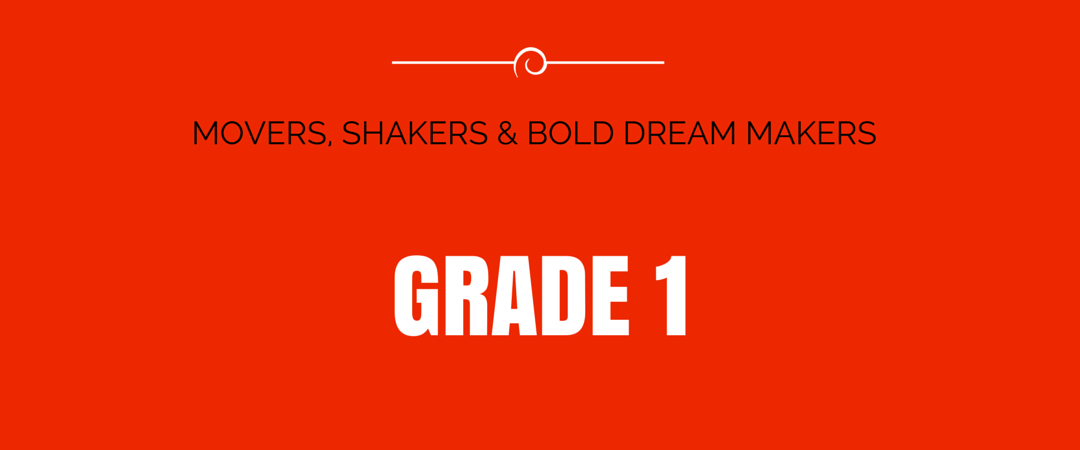#This year is a big year for reading. Your student will learn how to read poems and stories as well as describe characters, settings, and major events, using key details. Your student will:

• retell and summarize stories;
• recite the central message;
• describe characters, settings, and major events, using key details from the story
• learn to identify words and phrases that suggest feelings or appeal to the senses;
• explain the differences between fiction and nonfiction; and
• identify the narrator.

• Can you tell me what happened in the story at the beginning? What happened after that? What happened at the end of the story?
• Can you tell me where the story took place?
• Can you tell me the important things that happened in the story?
• Who are the characters in the story? What do you know about them?
• Can you find the feeling words in this poem/story?
• Is this book an informational book or a story book? What in the text leads you to that answer?
• Who is telling the story in this part of the book?
• Can you find an illustration or part that shows the main character?
• Can you find an illustration or part that shows the setting?
• Can you find an illustration or part that shows the problem in the story?
• What is the same about the characters in the two stories? What is different?
• What happened to the characters that is the same? What is different?
• Did the characters solve the problem in different ways? If so, how?

COMMON CORE EXPECTATIONS Your child should be able to:

• recite vowels;
• know many one syllable words;
• recognize individual sounds of combined letters;
• begin to break down syllables in two-syllable words;
• find words and phrases that point to feelings or senses;
• explain the difference between books that tell a story and books that provide information;
• identify who is telling the story at different points in the text;
• point to the book’s illustrations and details to describe characters, setting, or events;
• compare and contrast adventures and characters;
• recall key details;
• identify the main topic;
• find similarities and differences;
• describe connections between characters or events and ideas;
• clarify the meaning of grade-level words;
• distinguish types of information found in graphics and text;
• use basic capitalization, periods, and question marks;
• begin to learn to read and understand informational text;
• learn to gain meaning through context clues; and
• begin writing opinion, information paragraphs, and narrative that uses two appropriately sequenced events, some details, and has an introduction plus a conclusion.

Your student learns to add and subtract numbers from 0-20. Plus, this year brings an introduction to fractions, telling time, and deeper understanding of two-digit place value.

• work with numbers up to 120;
• learn to add and subtract numbers 0-20;
• begin to solve word problems using objects, drawings, and equations;
• measure lengths of an object;
• use shorter objects to build a new object of a desired length;
• learn to tell time;
• develop an understanding of two-digit place value, gaining competency in recognizing the ones and tens;
• begin to partition circles and rectangles into two and four equal shares; and
• recognize and describe halves and quarters of basic shapes.

• What time is it?
• How many of these small pieces will it take to measure this long?
• Can you divide this in half?
• Can you divide in quarters?
• How long is this?
• How many apples should we buy? How many more do we need to make that number?
• Would you please count the change and make sure it was correct?

COMMON CORE EXPECTATIONS
Your child should be able to:

• solve word problems using numbers 0-20. This will include adding to, taking from, putting together, taking apart, and comparing quantities by using objects, drawings and equations;
• solve word problems that call for addition of three whole numbers that add up to 20;
• show fluency for addition and subtraction for numbers 0-10;
• understand the equal sign;
• determine if equations involving addition and subtraction are true or false;
• determine the unknown whole number in an addition or subtraction;
• count to 120, starting at any number less than 120;
• understand that the digits of a two-digit number represent amounts of tens and ones;
• add to 100, including two-digit and one-digit numbers;
• add and subtract in multiples of 10, using models or drawings to show strategies based on place value, properties of operations, and/or the relationship between addition and subtraction;
• given a two-digit number, mentally find 10 more or 10 less;
• order three objects by length;
• compare lengths of two objects indirectly by using a third object;
• express the length of an object as a whole number of length units by laying multiple copies of a shorter object end to end;
• tell and write time in hours and half-hours using analog and digital clocks;
• understand and compare up to three categories of data;
• distinguish between defining attributes (e.g., triangles are closed and three-sided) versus non-defining attributes (e.g., color, orientation, overall size;
• compose two-dimensional shapes (rectangles, squares, trapezoids, triangles, half-circles, and quarter-circles) or three-dimensional shapes (cubes, right rectangular prisms, right circular cones, and right circular cylinders) to create a composite shape, and compose new shapes from the composite shape; and
• partition circles and rectangles into two and four equal shares.